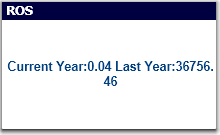# QlikView App Dev

Discussion Board for collaboration related to QlikView App Development.

cancel
Showing results for
Did you mean:Contributor III

## error in expression ')' expected

Hello,

i using the below expression to find the number of units sold. i'm getting a error saying (error in expression ')' expected ). can someone help me on this?

='Current Year:'& Num((Sum({<week = {">=\$(vMinDate)<=\$(vMaxdate)"}>} RTL_QTY)/Count(DISTINCT(week)),'##.00')
& ' Last Year:'&num((((Sum({<week = {">=\$(vRollingStart)<=\$(vRollingEnd)"},Period=>} RTL_QTY)/Count(DISTINCT(week))
-
Sum({<week = {">=\$(vRollingStart)<=\$(vRollingEnd)"},Period= {'LYTD'}> } RTL_QTY)/Count(DISTINCT(week))),'##.00')

4 RepliesMVP

Remove extra three braces after 2nd num function, like:

'Current Year:'& Num((Sum({<week = {">=\$(vMinDate)<=\$(vMaxdate)"}>} RTL_QTY)/Count(DISTINCT(week)),'##.00')
& ' Last Year:'&num((((Sum({<week = {">=\$(vRollingStart)<=\$(vRollingEnd)"},Period=>} RTL_QTY)/Count(DISTINCT(week))
-
Sum({<week = {">=\$(vRollingStart)<=\$(vRollingEnd)"},Period= {'LYTD'}> } RTL_QTY)/Count(DISTINCT(week))),'##.00')Contributor III
Author

i removed the brackets, still not working.MVP

I used:

``````='Current Year:'& Num((Sum({<week = {">=\$(vMinDate)<=\$(vMaxdate)"}>} RTL_QTY)/Count(DISTINCT( week))/1000000),'##.00')
& ' Last Year:'&num(Sum({<week = {">=\$(vRollingStart)<=\$(vRollingEnd)"},Period=>} RTL_QTY)/Count(DISTINCT( week))
-
Sum({<week = {">=\$(vRollingStart)<=\$(vRollingEnd)"},Period= {'LYTD'}> }RTL_QTY)/Count(DISTINCT( week)),'##.00')``````

and got:Contributor III
Author

the answer is not correct, it should be close to 1200,

if i select last year, then it shoould be retail quanity /52(number of weeks in a year). if i select current year from period filter, suppose current month is jan then calculation be like retail quantity/4(number of weeks in jan month) if current month is aug then calculation should be retail quanity/36(number weeks till august)  and if the current month is oct then calculation be like retail quantity/40(number of weeks till oct) .Community Browser